Homework Help Question & Answers

# Which factor(s) will influence the rate of a reaction? A. The kinetic energy of the molecules....

Which factor(s) will influence the rate of a reaction?

A. The kinetic energy of the molecules.

B. The number of molecules.

C. The physical state of the reactants.

D. All three of the answers shown.

#### Homework Answers

Answer #1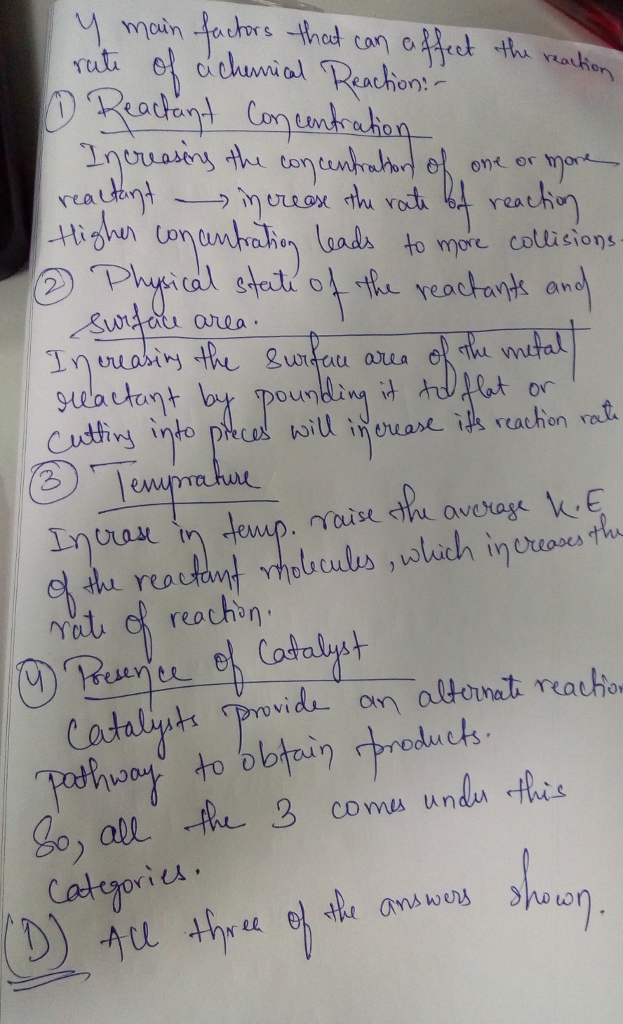Know the answer?
Your Answer:

#### Post as a guest

Your Name:

What's your source?

#### Earn Coin

Coins can be redeemed for fabulous gifts.

Not the answer you're looking for? Ask your own homework help question. Our experts will answer your question WITHIN MINUTES for Free.
Similar Homework Help Questions
• ### 12.) Which of the following factors influence the rate of a reaction? A) Temperature B) Reactant...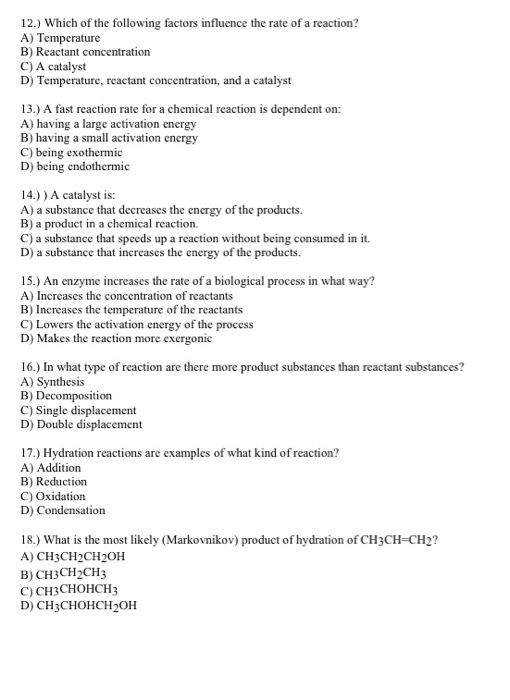12.) Which of the following factors influence the rate of a reaction? A) Temperature B) Reactant concentration C) A catalyst D) Temperature, reactant concentration, and a catalyst 13.) A fast reaction rate for a chemical reaction is dependent on: A) having a large activation energy B) having a small activation energy C) being exothermic D) being endothermic 14.)) A catalyst is A) a substance that decreases the energy of the products. B) a product in a chemical reaction. c) a...

• ### Kinetics Broadly speaking, the factors that influence chemical reaction rates may be summarised as follows. Chemical...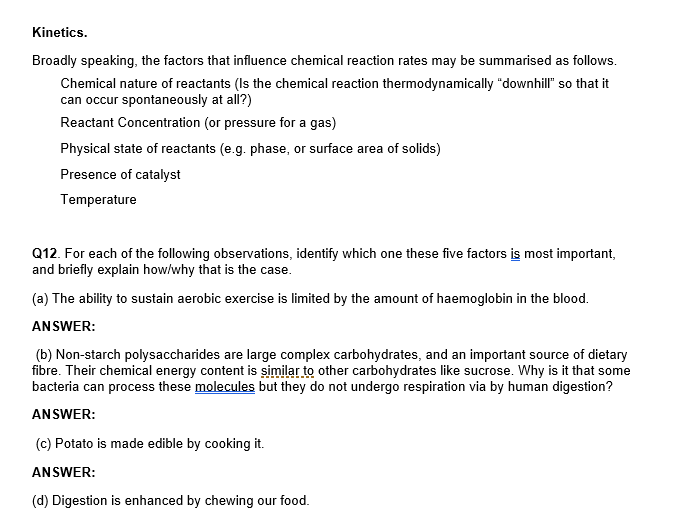Kinetics Broadly speaking, the factors that influence chemical reaction rates may be summarised as follows. Chemical nature of reactants (Is the chemical reaction thermodynamically "downhill so that it can occur spontaneously at all?) Reactant Concentration (or pressure for a gas) Physical state of reactants (e.g. phase, or surface area of solids) Presence of catalyst Temperature 012 For each offervations, identify which one these five factors is most important, and briefly explain how/why that is the case. (a) The ability to...

• ### CHEMISTRY EXPERT ONLY PLEASE WORK ON THE TABLE. I WILL RATE THANK YOU CHM 1046 Workbook...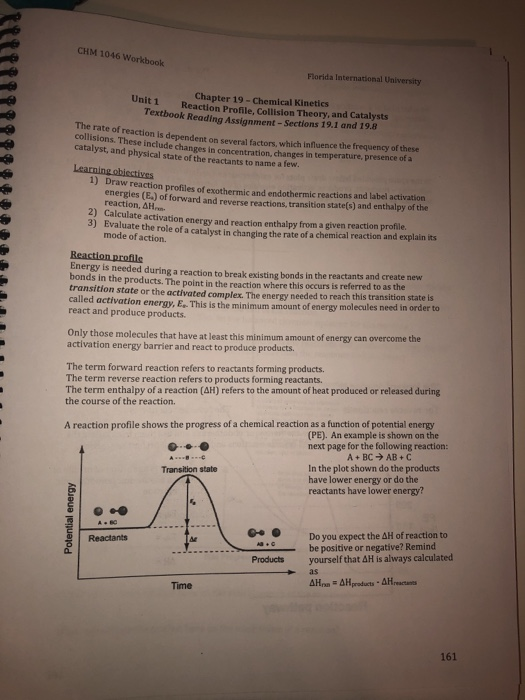CHEMISTRY EXPERT ONLY PLEASE WORK ON THE TABLE. I WILL RATE THANK YOU CHM 1046 Workbook Florida International University Chapter 19-Chemical Kinetics Reaction Profile, Collision Theory, and Catalysts Textbook Reading Assignment-Sections 19.1 and 19.8 The rate of reaction is dependent on several factors, which influence the frequency of these collisions. These include changes in concentration, changes in temperature, presence of a Unit 1 catalyst, and physical state of the reactants to name a few. Learning obiectives 1) Draw reaction profiles...

• ### What factor affects the rate of a chemical reaction? collision frequency orientation of molecules fraction of...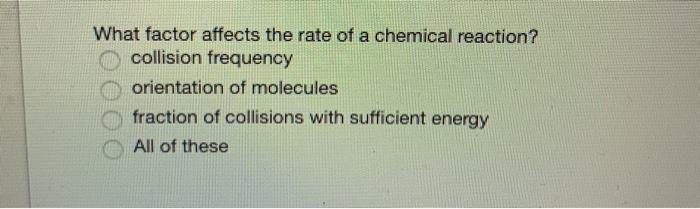What factor affects the rate of a chemical reaction? collision frequency orientation of molecules fraction of collisions with sufficient energy All of these

• ### Thank you everymuch I will rate quickly when answered! Chemical reactions occur when molecules or atoms...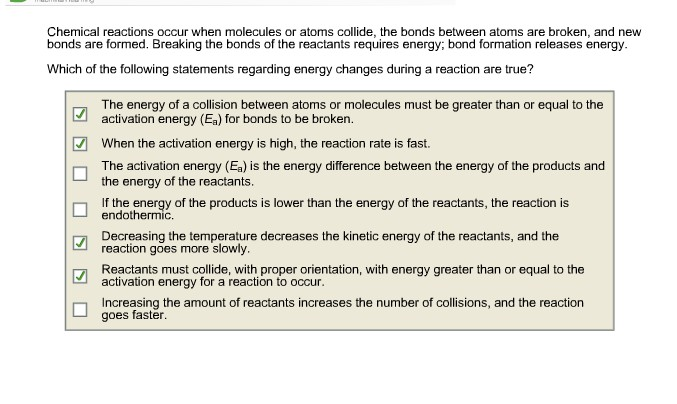Thank you everymuch I will rate quickly when answered! Chemical reactions occur when molecules or atoms collide, the bonds between atoms are broken, and new bonds are formed. Breaking the bonds of the reactants requires energy; bond formation releases energy Which of the following statements regarding energy changes during a reaction are true? The energy of a collision between atoms or molecules must be greater than or equal to the activation energy (Ea) for bonds to be broken. When the...

• ### ENED NERGY VS REACTION COORDINATE DIAGRAM MODEL T ction coordinate graph is used to show how...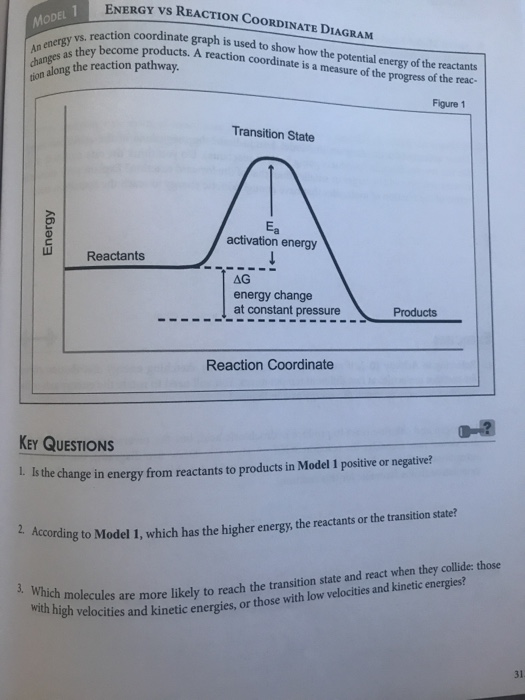ENED NERGY VS REACTION COORDINATE DIAGRAM MODEL T ction coordinate graph is used to show how the potential energy of the reactants become products. A reaction coordinate is a measure of the progress of the reac- An energy vs. reaction changes as they becom along the reaction pathway. Figure 1 Transition State Energy activation energy Reactants AG energy change at constant pressure Products Reaction Coordinate KEY QUESTIONS 1. Is the change in energy from reactants to products in Model 1...

• ### 1.. Which statement is correct about the changes in potential energy and kinetic energy when two...

1.. Which statement is correct about the changes in potential energy and kinetic energy when two N atoms combine to form an N2 molecule? Group of answer choices a. The potential energy becomes more negative and the kinetic energy increases. b. The potential energy becomes more positive and the kinetic energy decreases. c. The potential energy becomes more positive and the kinetic energy increases. d. The potential energy becomes more negative and the kinetic energy decreases. 2.. You take solid...

• ### To use the Arrhenius equation to calculate the activation energy. As temperature rises, the average kinetic...

To use the Arrhenius equation to calculate the activation energy. As temperature rises, the average kinetic energy of molecules increases. In a chemical reaction, this means that a higher percentage of the molecules possess the required activation energy, and the reaction goes faster. This relationship is shown by the Arrhenius equation k=Ae−Ea/RT where k is the rate constant, A is the frequency factor, Ea is the activation energy, R = 8.3145 J/(K⋅mol) is the gas constant, and T is the...

• ### Question 15 Identify correct statements pertaining to reaction energies. The rate of a reaction depends on...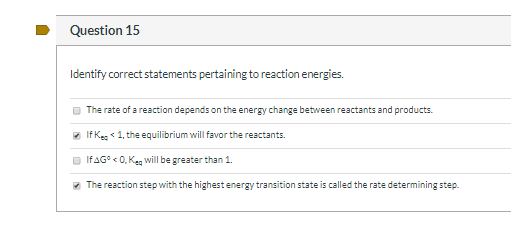Question 15 Identify correct statements pertaining to reaction energies. The rate of a reaction depends on the energy change between reactants and products, If Keo < 1, the equilibrium will favor the reactants. IfAG® < 0. Ke will be greater than 1. The reaction step with the highest energy transition state is called the rate determining step. Which of the compounds shown below are meso? (choose all correct answers) HO.

• ### Which of the following is not a factor involved in determining the rate of a chemical...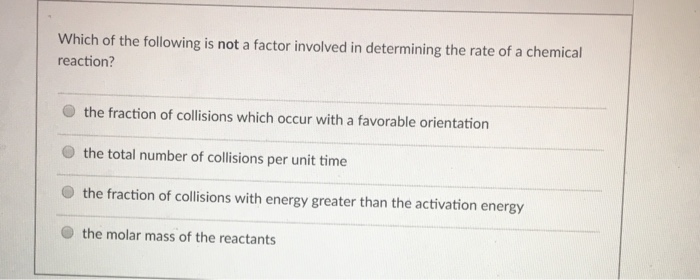Which of the following is not a factor involved in determining the rate of a chemical reaction? the fraction of collisions which occur with a favorable orientation the total number of collisions per unit time the fraction of collisions with energy greater than the activation energy the molar mass of the reactants